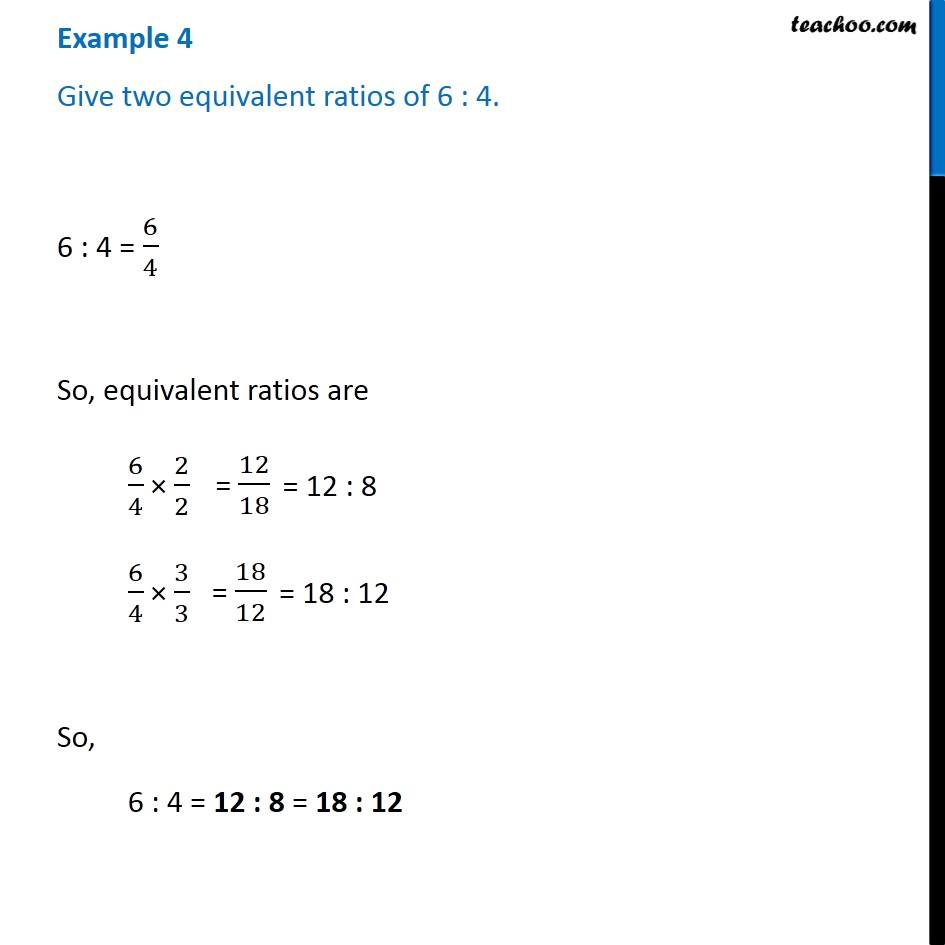1. Chapter 12 Class 6 Ratio And Proportion
2. Concept wise
3. Equivalent ratios

Transcript

Example 4 Chapter 12 Class 6 Give two equivalent ratios of 6 : 4. 6 : 4 = 6/4 So, equivalent ratios are 6/4 × 2/2 = 12/8 = 12:8 6/4 × 3/3 = 18/12 = 18:12 So, 6 : 4 = 12 : 8 = 18 : 12